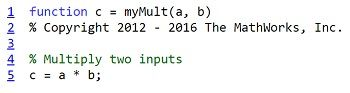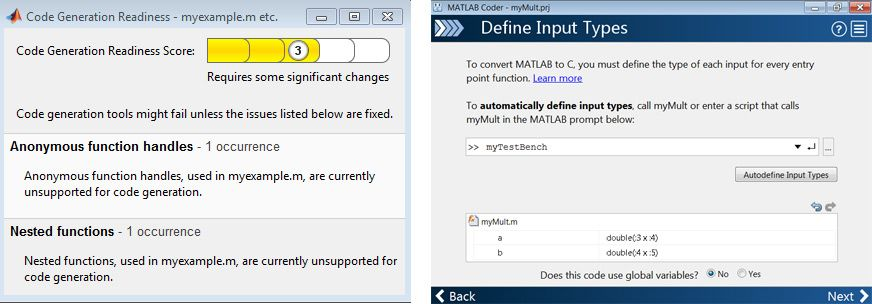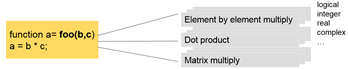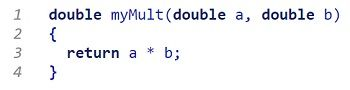# The Joy of Generating C Code from MATLAB

By Bill Chou, MathWorks

Engineers have translated low-level languages like C into machine code for decades using compilers. But is it possible to translate a high-level language like MATLAB® to C using coders? Most engineers would agree that it’s possible in theory—but does it work in practice? Is the generated code readable or spaghetti? Efficient or bloated? Fast or slow? And does it support industrial workflows, or just R&D?

This article addresses these concerns head-on. It provides tips and best practices for working with MATLAB Coder™, as well as industry examples of successful applications of generated code by companies such as Delphi, Baker Hughes, iSonea, and dorsaVi.

## Comparing MATLAB and C Code: A Multiplication Example

The simple MATLAB function below multiplies two inputs.Given scalar inputs, MATLAB Coder generates the following C code:As you can see, the generated code maps clearly back to the MATLAB code.

The same piece of MATLAB code, when given two matrix inputs, generates three nested `for`-loops in C:## Recommended Three-Step Iterative Workflow

The simple function shown above can be implemented in a single step. But for more substantial projects, we recommend a structured approach using a three-step iterative workflow (Figure 1):

1. Prepare your algorithm for code generation. Examine and modify the MATLAB code to introduce implementation considerations needed for low-level C code, and use the MATLAB language and functions that support code generation.
2. Test the MATLAB code’s readiness for code generation using default settings. Check for run-time errors by generating and executing a MEX file. If successful, move to the next step. If not, repeat step 1 until you can generate a MEX function.
3. Generate C code or keep the MEX function from step 2. You can iterate on the MATLAB code to optimize either the generated C code (for look and feel, memory, and speed) or the MEX function (for performance).Figure 1. Three-step iterative workflow for generating code.

The MATLAB Coder app guides you through this iterative process while enabling you to stay within the MATLAB environment. It analyzes your MATLAB code to propose data types and sizes for your inputs. It tests whether your MATLAB code is ready for code generation by generating a MEX function, then executes the MEX function to check for run-time errors (Figure 2). Equivalent command-line functions provide the same functionality so you can generate code as part of a script or function.Figure 2. Left: Automated checks for features and functions not supported for code generation. Right: Automated analysis and proposal for input data types and sizes.

The video below illustrates these steps with an example of generating a Kalman filter to predict the trajectory of a bouncing ball. You’ll see that the three-step iterative process enables us to generate code that closely matches the original MATLAB results and satisfies its tracking requirements.## Implementation Constraints

As you prepare your MATLAB algorithm for code generation, you need to take account of implementation constraints resulting from the differences between MATLAB and C code. These include:

• Memory allocation. In MATLAB, memory allocation is automatic. In C code, memory allocation is manual—it is allocated either statically (using `static`), dynamically (using `malloc`), or on the stack (using local variables).
• Array-based language. MATLAB provides a rich set of array operations that allow concise coding of numerical algorithms. C code requires explicit `for`-loops to express the same algorithms.
• Dynamic typing. MATLAB automatically determines the data types and sizes as your code runs. C requires explicit type declarations on all variables and functions.
• Polymorphism. MATLAB functions can support many different input types, while C requires fixed type declarations. At the top level, you must specify the intended C function declaration.

Let’s take a closer look at polymorphism. Polymorphism can give a single line of MATLAB code different meanings depending on your inputs. For example, the function shown in Figure 3 could mean scalar multiplication, dot product, or matrix multiplication. In addition, your inputs could be of different data types (logical, integer, floating-point, fixed-point), and they could be real or complex numbers.Figure 3. Polymorphism example.

MATLAB is a powerful algorithm development environment precisely because you don’t need to worry about implementation details as you create algorithms. However, for the equivalent C code, you have to specify what operations mean. For example, the line of MATLAB code shown above could be translated into this single line of C code that returns B*C:Or, it could be translated into 11 lines of C code with 3 `for`-loops that multiply two matrices:The video below uses a Newton-Raphson algorithm to illustrate the concept of taking implementation constraints into account. You’ll see that code generated using the three-step iterative workflow exactly matches the original MATLAB results.## Working with the Generated Code: Four Use Cases

Once you have generated readable and portable C/C++ code from MATLAB algorithms using MATLAB Coder, you have several options for using it. For example, you can:

## Industry Success Stories

• Baker Hughes’ Dynamics & Telemetry group generated a DLL from sequence prediction algorithms and integrated it into surface decoding software running on a PC that enables downhole data to be decoded quickly and reliably during drilling operations.
• dorsaVi generated C++ code from motion analysis algorithms and compiled it into a DLL, which was then integrated into their C# application running on a PC that analyzes the athlete’s movements to diagnose injury.

• VivaQuant generated fixed-point C code from heart rhythm monitoring algorithms and compiled it for an ARM Cortex-M processor.
• Delphi generated C code for an automotive radar sensor alignment algorithm and compiled it for an ARM10 processor.
• Respiri generated C code from acoustic respiratory monitoring algorithms and compiled it for an iPhone app, an Android app, and cloud-based server software.

## Multicore-Capable Code Generation and Other Optimization Methods

In MATLAB, `for`-loops whose iterations are independent of each other can be run in parallel simply by replacing `for` with `parfor`. MATLAB Coder uses the Open Multiprocessing (OpenMP) application interface to support shared-memory, multicore code generation from `parfor` loops. OpenMP is supported by many C compilers (for example, Microsoft® Visual Studio® Professional).You can use MATLAB Coder with Embedded Coder® to further optimize code efficiency and customize the generated code. Embedded Coder provides optimizations for fine-grained control of the generated code’s functions, files, and data. For example, you can use storage classes to control the declaration and definition of a global variable in the generated code, and use code generation templates to customize banners and comments in the generated code. Embedded Coder also improves code efficiency by using code replacement libraries, which replace certain operators and functions with implementations optimized for popular processors like ARM Cortex®-A and ARM Cortex-M.

## Testing the Generated Code

As you develop your MATLAB algorithm, you can create unit tests to verify that the algorithm produces the results you expect. Tests written using the MATLAB unit testing framework can be reused to verify that the generated code behaves the same way as your MATLAB algorithm. The videos below show how you can reuse the unit tests in Embedded Coder in combination with software-in-the-loop (SIL) and processor-in-the-loop (PIL) tests on the generated standalone code or library (Figure 4).## An Automated Workflow

MATLAB Coder enables an automated workflow for translating MATLAB algorithms into C code. With this workflow you spend less time writing and debugging low-level C code and more time developing, testing, and tuning designs. By maintaining one golden reference in MATLAB, including the algorithm and test benches, you can propagate algorithmic changes to your C code more quickly. Automated tools like the MATLAB unit testing framework and the Embedded Coder SIL and PIL testing framework let you test both the MATLAB code and the C code thoroughly and systematically. Whether you are implementing designs running on traditional PCs, web servers, mobile devices, or embedded processors, MATLAB Coder will help you get from MATLAB to C code faster and with fewer manual translation errors.

Published 2016 - 92987v00